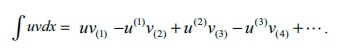Home | | Maths 12th Std | Summary

# Summary

Maths : Applications of Integration: Summary

SUMMARY

### (1) Definite integral as the limit of a sum### (2) Properties of definite integrals### (3) BernoulliŌĆÖs Formula### (4) Reduction Formulae### (5) Gamma Formulae### (6) Area of the region bounded by a curve and lines

(i) The area of the region bounded by a curve, above x -axis and the lines x = a and x = b is A = bŌł½a y dx .

(ii) The area of the region bounded by a curve, below x -axis and the lines x = a and x = b is A = ŌłÆbŌł½a ydx = | bŌł½a ydx | .

(iii) Thus area of the region bounded by the curve to the right of y -axis, the lines y = c and y = d is A = dŌł½c  xdy .

(iv) The area of the region bounded by the curve to the left of y -axis, the lines y = c and y = d is A = ŌłÆ dŌł½c xdy = | dŌł½c xdy |.

(v) If fŌĆē(x) Ōēź g(x), then area bounded by the curves and the lines x = a, x = b is

A = bŌł½a [ f (x) ŌłÆ g( x)]dx = bŌł½a ( yU ŌłÆ yL ) dx

(vi) If fŌĆē(y) Ōēź g(y), then area bounded by the curves and the lines y = c, y = d is

A = dŌł½c [ f ( y) ŌłÆ g(y)]dy = dŌł½c ( xR ŌłÆ xL ) dy

### (7) Volume of the solid of revolution

(i) The volume of the solid of revolution about x-axis is V = ŽĆ bŌł½a y2 dx.

(ii) The volume of the solid of revolution about y-axis is V = ŽĆ dŌł½c x2 dy.

Tags : Applications of Integration | Mathematics , 12th Maths : UNIT 9 : Applications of Integration
Study Material, Lecturing Notes, Assignment, Reference, Wiki description explanation, brief detail
12th Maths : UNIT 9 : Applications of Integration : Summary | Applications of Integration | Mathematics